# Linear Algebra Chapter 6 Linear Algebra with Applications

• Slides: 11Linear Algebra Chapter 6 Linear Algebra with Applications -Gareth Williams Br. Joel Baumeyer, F. S. C.Definition: Eigenvalue & Eigenvector n n The Characteristic Polynomial of Anxn: |A - I| The Characteristic Equation of Anxn: |A - I| = 0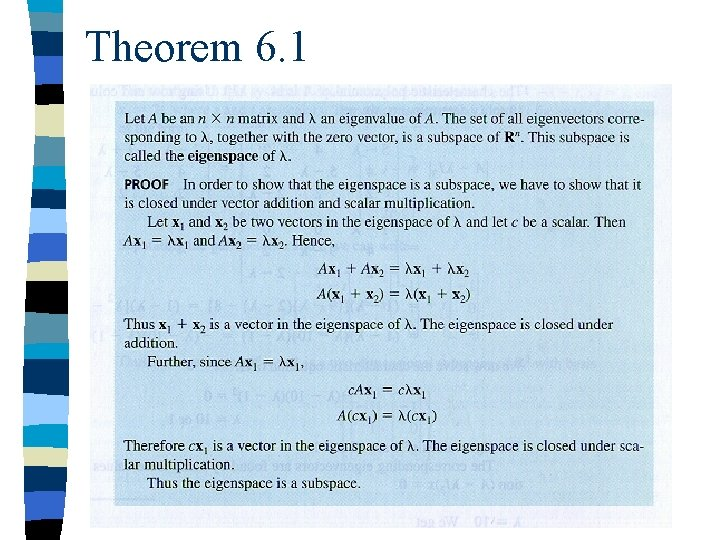Theorem 6. 1Similar Matrices n Definition: Let A and B be square matrices of the same size. B is said to be similar to A if there exists an invertible matrix C such that B = C-1 AC. The transformation of the matrix A into the matrix B in this manner is called a similarity transformation.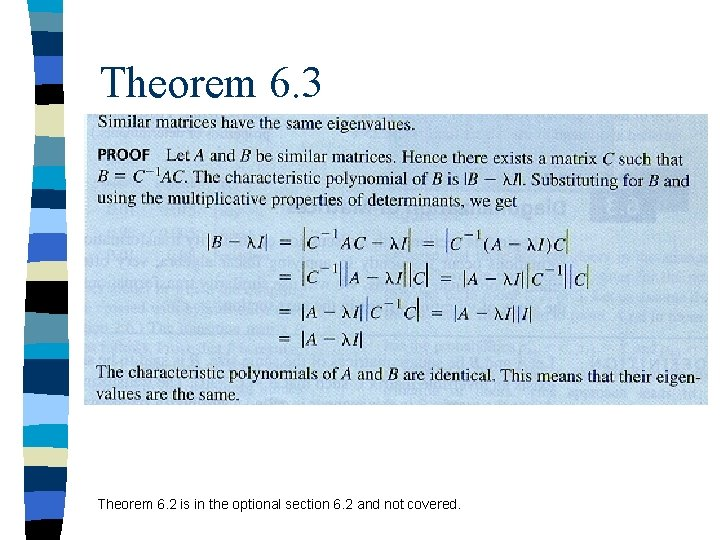Theorem 6. 3 Theorem 6. 2 is in the optional section 6. 2 and not covered.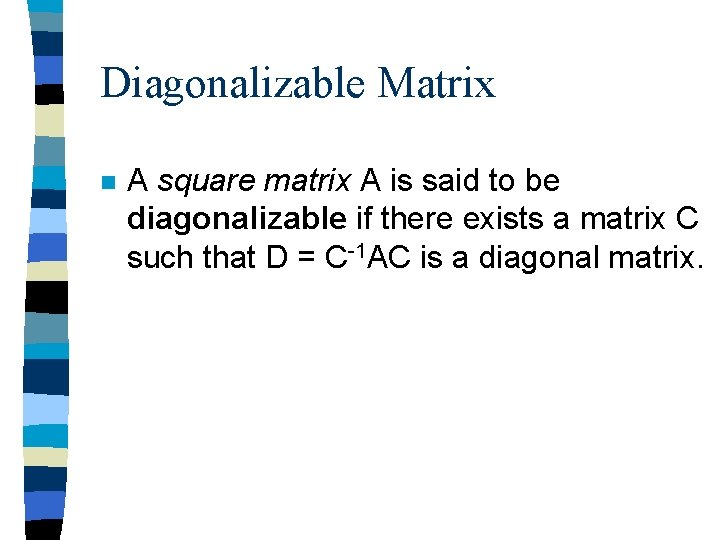Diagonalizable Matrix n A square matrix A is said to be diagonalizable if there exists a matrix C such that D = C-1 AC is a diagonal matrix.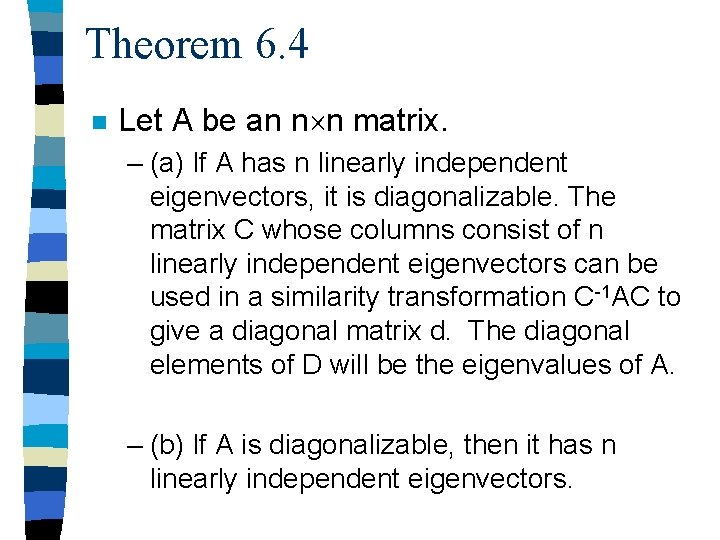Theorem 6. 4 n Let A be an n n matrix. – (a) If A has n linearly independent eigenvectors, it is diagonalizable. The matrix C whose columns consist of n linearly independent eigenvectors can be used in a similarity transformation C-1 AC to give a diagonal matrix d. The diagonal elements of D will be the eigenvalues of A. – (b) If A is diagonalizable, then it has n linearly independent eigenvectors.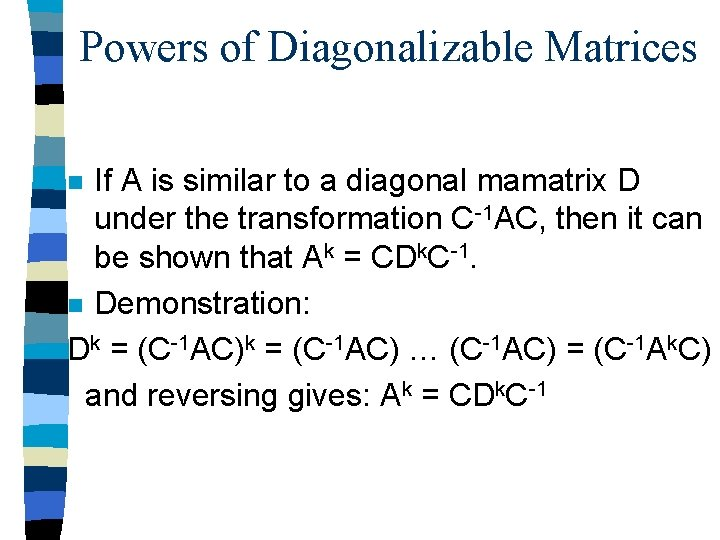Powers of Diagonalizable Matrices If A is similar to a diagonal mamatrix D under the transformation C-1 AC, then it can be shown that Ak = CDk. C-1. n Demonstration: Dk = (C-1 AC) … (C-1 AC) = (C-1 Ak. C) and reversing gives: Ak = CDk. C-1 nTheorem 6. 5 n Let a be an nxn symmetrix matrix. – a) All the eigenvalues of A are real numbers. – b) the dimension of an eigenspace of A is the multiplicity of the eigenvalue as a root of the characteristic equation. – c) The eigenspaces of A are orthogonal. – d)A has n linearly independent eigenvectors.Orthogonally Diagonalizable Definition: A square matrix A is said to be orthogonally diagonalizable if there exists an ortholgonal matrix C such that D = Ct. AC is a diagonal matrix. nTheorem 6. 6 n Let a be a square matrix. A is orthogonally diagonalizable if and only if it is a symmetric matrix.